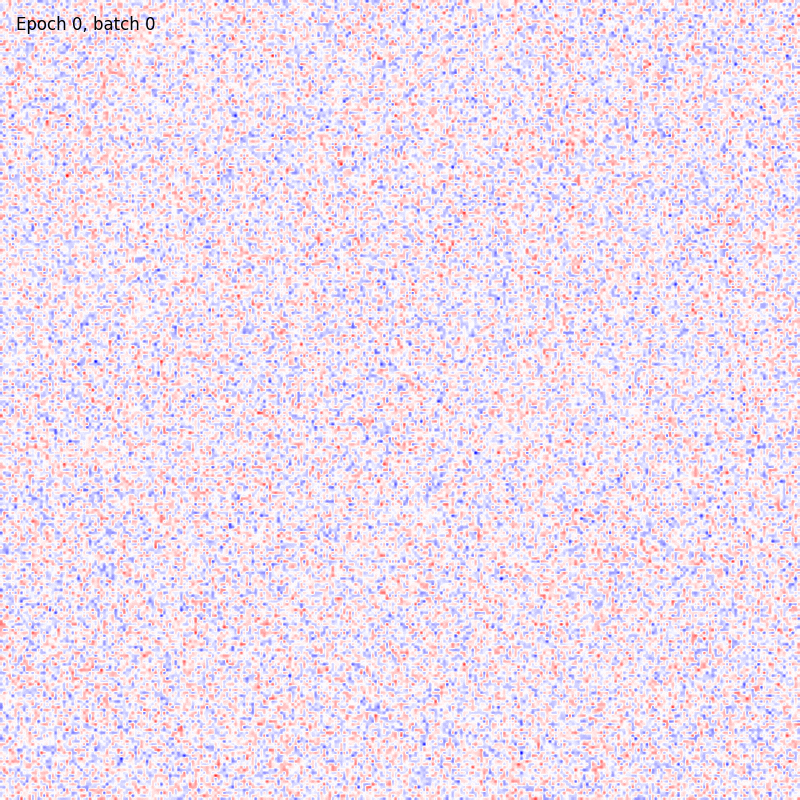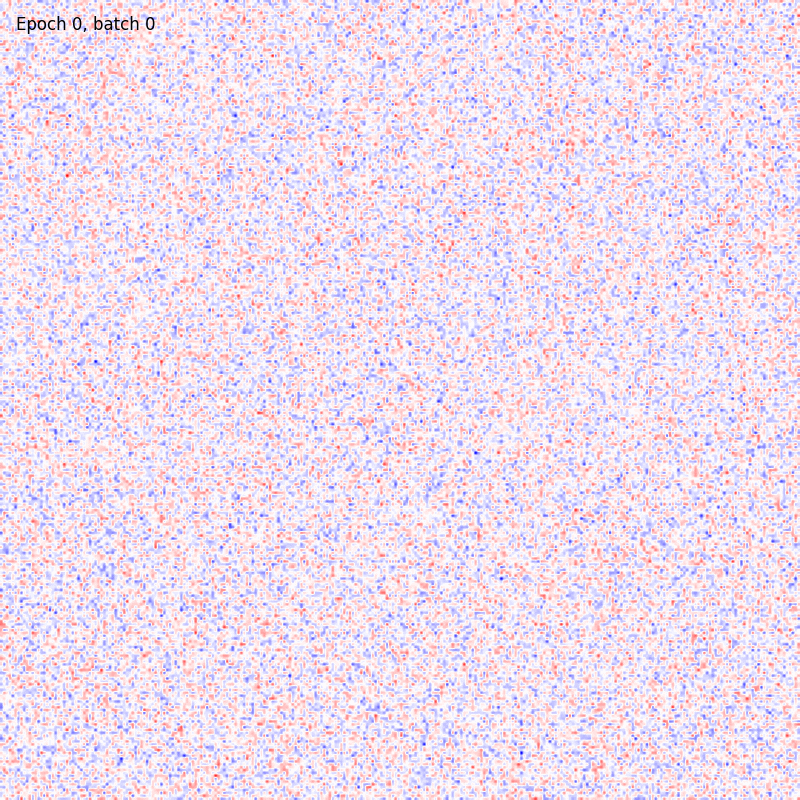# Usage example¶

You can use the plasticity library into pure-Python modules or inside your C++ application.

## Python example¶

The plasticity classes are totally equivalent to a scikit-learn feature-encoder method and thus they provide the member functions fit (to train your model) and predict (to test a trained model on new samples).

First of all you need to import the desired plasticity class and then simply call the training/testing functions.

from plasticity.models import BCM
from sklearn.datasets import fetch_openml

X, y = fetch_openml(name='mnist_784', version=1, data_id=None, return_X_y=True)

# normalize the sample into [0, 1]
X *= 1. / 255

from plasticity.model import BCM

model = BCM(outputs=100, num_epochs=10, batch_size=100, interaction_strenght=0.)
model.fit(X)


You can also visualize the weights connections using the utility functions provided by the package as

from plasticity.utils import view_weights

view_weights (model.weights, dims=(28, 28))


The results should be something likeFor sake of completeness the same pipeline can be run also with the Hopfield model. In this case the could will be

from plasticity.models import Hopfield
from sklearn.datasets import fetch_openml

X, y = fetch_openml(name='mnist_784', version=1, data_id=None, return_X_y=True)

# normalize the sample into [0, 1]
X *= 1. / 255

from plasticity.model import Hopfield

model = Hopfield(outputs=100, num_epochs=10, batch_size=100)
model.fit(X)


And in this case the weights matrix should appear similar to